This Rust implementation embodies the latest implementation Adapton, which offers a foundational, language-based semantics for general-purpose incremental computation.

## Background

Adapton proposes the demanded computation graph (or DCG), and a demand-driven change propagation algorithm. Further, it proposes first-class names for identifying cached data structures and computations.

The following academic papers detail these technical proposals:

## Why Rust?

Adapton's first implementations used Python and OCaml; The latest implementation in Rust offers the best performance thus far, since (1) Rust is fast, and (2) traversal-based garbage collection presents performance challenges for incremental computation. By liberating Adapton from traversal-based collection, our empirical results are both predictable and scalable.

• The Editor role creates and mutates input, and demands the output of incremental computations in the Archivist role.

• The Archivist role consists of Adapton thunks, where each is cached computation that consumes incremental input and produces incremental output.

Examples: The examples below illustrate these roles, in increasing complexity:

Programming primitives: The following list of primitives covers the core features of the Adapton engine. Each primitive below is meaningful in each of the two, editor and archivist, roles:

# Start the DCG engine

The call `init_dcg()` below initializes a DCG-based engine, replacing the `Naive` default engine.

```#[macro_use] extern crate adapton;

fn main() {
manage::init_dcg();

// Put example code below here
}```

# Create incremental cells

Commonly, the input and intermediate data of Adapton computations consists of named reference `cell`s. A reference `cell` is one variety of `Art`s; another are `thunk`s.

## Implicit counter for naming `cell`s

`cell!(123)` uses a global counter to choose a unique name to hold `123`. Important note: This may be appopriate for the Editor role, but is never appropriate for the Archivist role.

```let c : Art<usize> = cell!( 123 );

assert_eq!( get!(c), 123 );```

## Explicitly-named `cell`s

Sometimes we name a cell using a Rust identifier. We specify this case using the notation `[ name ]`, which specifies that the cell's name is a string, constructed from the Rust identifer `name`:

```let c : Art<usize> = cell!([c] 123);

assert_eq!(get!(c), 123);```

## Optionally-named `cell`s

Most generally, we supply an expression `optional_name` of type `Option<Name>` to specify the name for the `Art`. This `Art` is created by either `cell` or `put`, in the case that `optional_name` is `Some(name)` or `None`, respectively:

```let n : Name = name_of_str(stringify!(c));
let c : Art<usize> = cell!([Some(n)]? 123);

assert_eq!(get!(c), 123);

let c = cell!([None]? 123);

assert_eq!(get!(c), 123);```

# Observe `Art`s

The macro `get!` is sugar for `engine::force!`, with reference introduction operation `&`:

```let c : Art<usize> = cell!(123);

assert_eq!( get!(c), force(&c) ); ```

Since the type `Art<T>` classifies both `cell`s and `thunk`s, the operations `force` and `get!` can be used interchangeably on `Art<T>`s that arise as `cell`s or `thunk`s.

# Mutate input cells

One may mutate cells explicitly, or implicitly, which is common in Nominal Adapton.

The editor (implicitly or explicitly) mutates cells that hold input and they re-demand the output of the archivist's computations. During change propagation, the archivist mutates cells with implicit mutation.

Implicit mutation uses nominal allocation: By allocating a cell with the same name, one may overwrite cells with new content:

```let n : Name = name_of_str(stringify!(c));
let c : Art<usize> = cell!([Some(n.clone())]? 123);

assert_eq!(get!(c), 123);

// Implicit mutation (re-use cell by name `n`):
let d : Art<usize> = cell!([Some(n)]? 321);

assert_eq!(d, c);
assert_eq!(get!(c), 321);
assert_eq!(get!(d), 321);```

No names implies no effects: Using `None` to allocate cells always **gives distinct cells, with no overwriting:

```
let c = cell!([None]? 123);
let d = cell!([None]? 321);

assert_eq!(get!(c), 123);
assert_eq!(get!(d), 321);```

Explicit mutation, via `set`: If one wants mutation to be totally explicit, one may use `set`:

```let n : Name = name_of_str(stringify!(c));
let c : Art<usize> = cell!([Some(n)]? 123);

assert_eq!(get!(c), 123);

// Explicit mutation (overwrites cell `c`):
set(&c, 321);

assert_eq!(get!(c), 321);```

# Demand-driven change propagation

The example below demonstrates demand-driven change propagation, which is unique to Adapton's approach to incremental computation. It employs two mutable input `cell`s, and two `thunk`s.

Thunks consist of computations whose observations and results are cached in the DCG.

The example's two mutable inputs, `num` and `den`, feed into an intermediate subcomputation `div` that divides the numerator in `num` by the denominator in `den`, and a thunk `check` that first checks whether the denominator is zero (returning zero if so) and if non-zero, returns the value of the division.

```// Two mutable inputs, for numerator and denominator of division
let num = cell!(42);
let den = cell!(2);

// In Rust, cloning is explicit:
let den2 = den.clone(); // clone _global reference_ to cell.
let den3 = den.clone(); // clone _global reference_ to cell, again.

// Two subcomputations: The division, and a check thunk with a conditional expression
let div   = thunk![ get!(num) / get!(den) ];
let check = thunk![ if get!(den2) == 0 { None } else { Some(get!(div)) } ];

// Observe output of `check` while we change the input `den`
// Step 1: (Explained in detail, below)
assert_eq!(get!(check), Some(21));

// Step 2: (Explained in detail, below)
set(&den3, 0);
assert_eq!(get!(check), None);

// Step 3: (Explained in detail, below)
set(&den3, 2);
assert_eq!(get!(check), Some(21));  // division is reused```

The programmer's changes and observations in the last lines induce the following change propagation behavior:

1. When the `check` is demanded the first time, it executes the condition, and `den` holds `2`, which is non-zero. Hence, the `else` branch executes `get!(div)`, which demands the output of the division, `21`.

2. After this first observation of `check`, the programmer changes `den` to `0`, and re-demands the output of `check`. In response, change propagation first re-executes the condition (not the division), and the condition branches to the `then` branch, resulting in `None`; in particular, it does not re-demand the `div` node, though this node still exists in the DCG.

3. Next, the programmer changes `den` back to its original value, `2`, and re-demands the output of `check`. In response, change propagation re-executes the condition, which re-demands the output of `div`. Change propagation attempts to "clean" the `div` node before re-executing it. To do so, it compares its last observations of `num` and `den` to their current values, of `42` and `2`, respectively. In so doing, it finds that these earlier observations match the current values. Consequently, it reuses the output of the division (`21`) without having to re-execute the division.

Slides with illustrations of the graph structure and the code side-by-side may help:

Step 1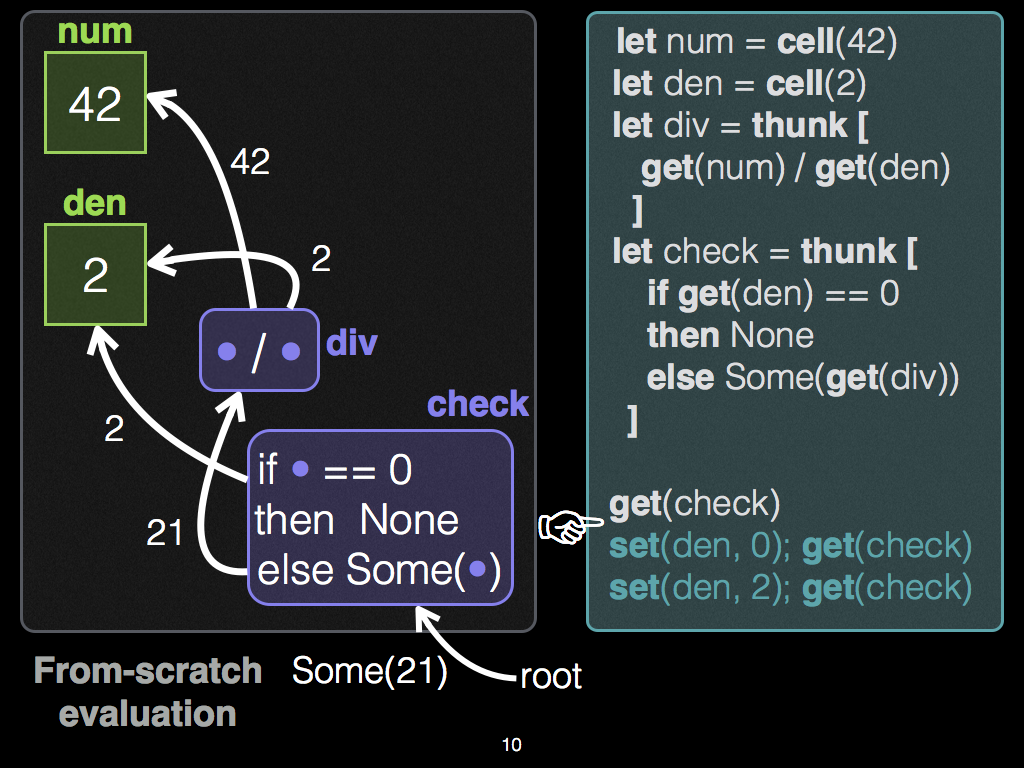Steps 2 and 3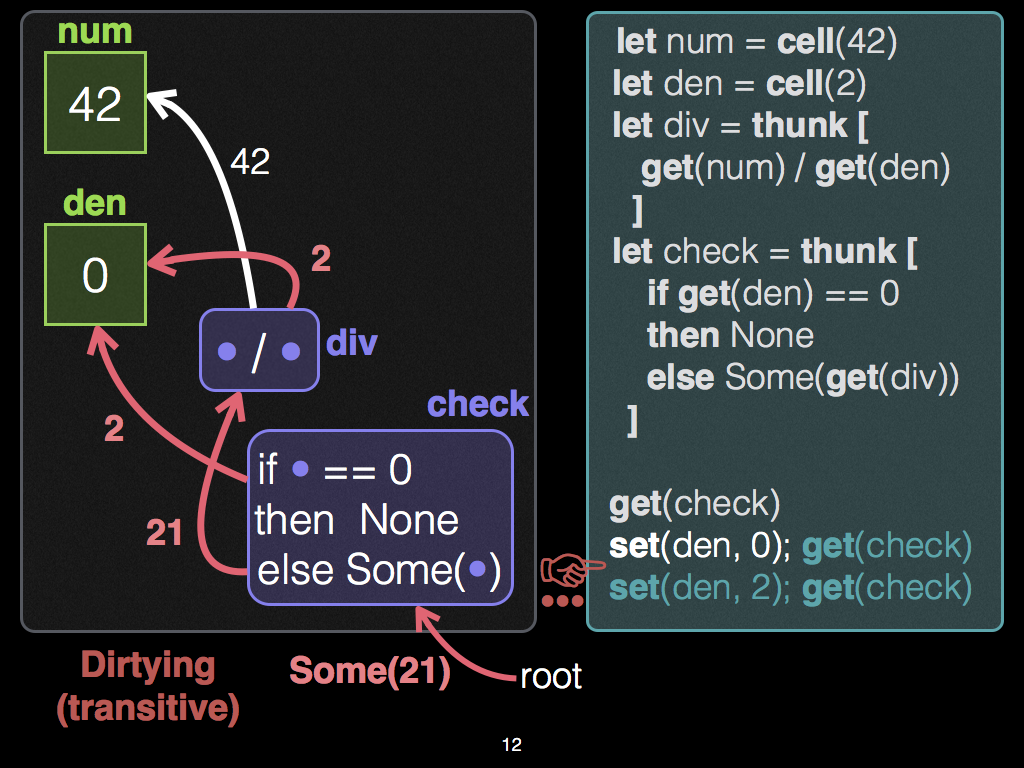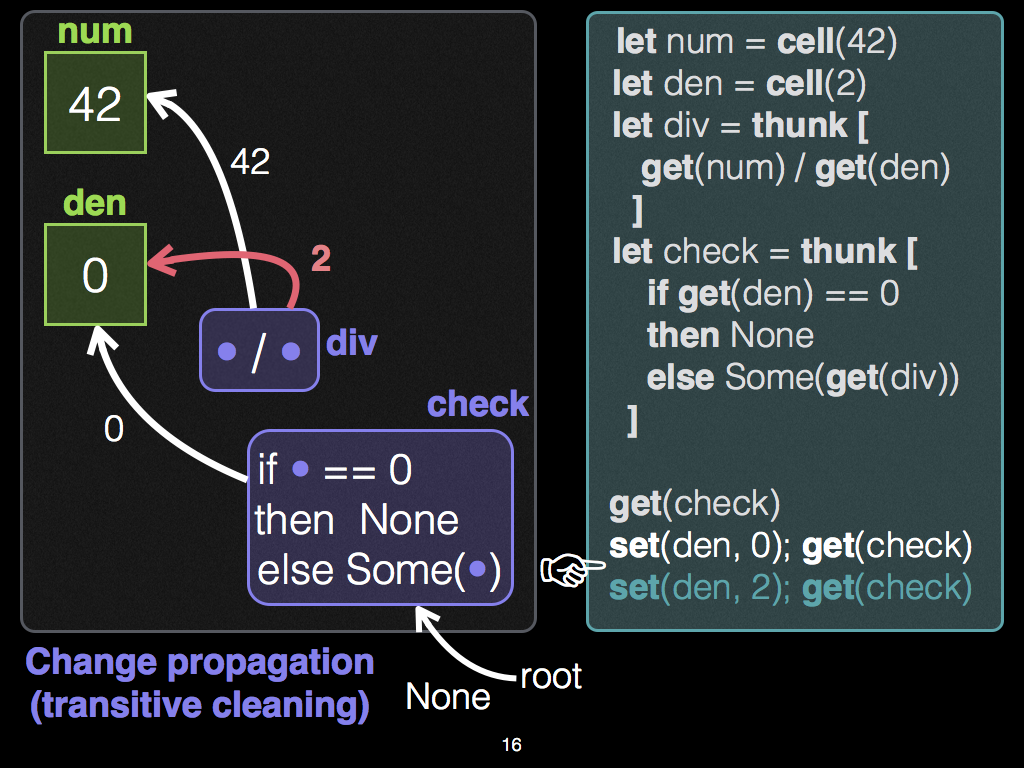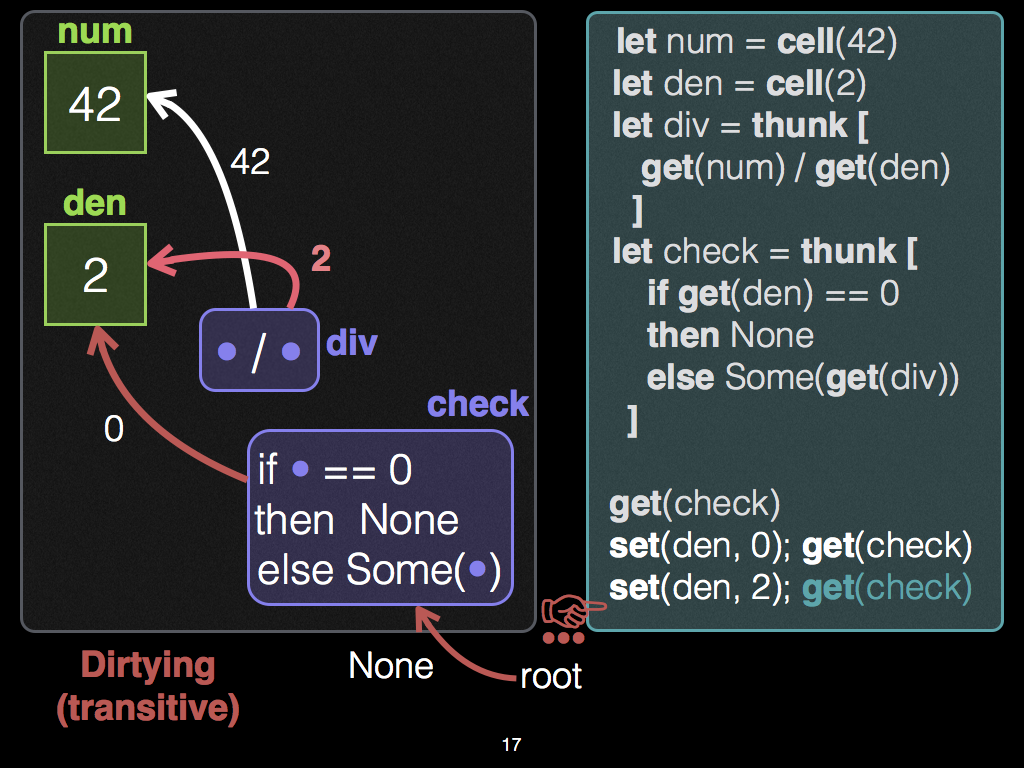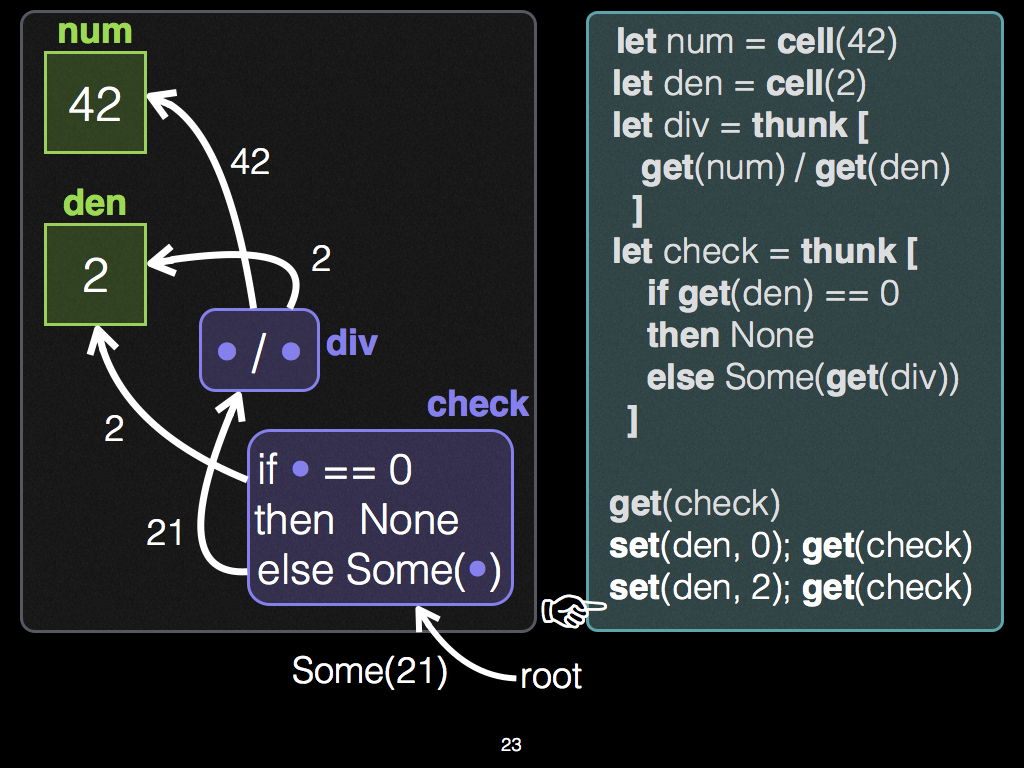Full-sized slides

## Switching

In the academic literature on Adapton, we refer to the three-step pattern of change propagation illustrated above as switching:

1. The demand of `div` switches from being present (in step 1),
2. to absent (in step 2),
3. to present (in step 3).

Past work on self-adjusting computation does not support this switching pattern directly: Because of its change propagation semantics, it would "forget" the division in step 2, and rerun it from-scratch in step 3.

Furthermore, some other change propagation algorithms base their re-execution schedule on "node height" (of the graph's topological ordering). These algorithms may also have undesirable behavior. In particular, they may re-execute the division in step 2, though it is not presently in demand. For an example, see this gist.

# Memoization

Memoization provides a mechanism for caching the results of subcomputations; it is a crtical feature of Adapton's approach to incremental computation.

In Adapton, each memoization point has three ingredients:

• A function expression (of type `Fn`)

• Zero or more arguments. Each argument type must have an implementation for the traits `Eq + Clone + Hash + Debug`. The traits `Eq` and `Clone` are both critical to Adapton's caching and change propagation engine. The trait `Hash` is required when Adapton's naming strategy is structural (e.g., where function names are based on the hashes of their arguments). The trait `Debug` is useful for debugging, and reflection.

• An optional name, which identifies the function call for reuse later.

• When this optional name is `None`, the memoization point may be treated in one of two ways: either as just an ordinary, uncached function call, or as a cached function call that is identified structurally, by its function pointer and arguments. Adapton permits structural subcomputations via the engine's structural function.

• When this is `Some(name)`, the memoization point uses `name` to identify the work performed by the function call, and its result. Critically, in future incremental runs, it is possible for `name` to associate with different functions and/or argument values.

Each memoization point yields two results:

• A thunk articulation, of type `Art<Res>`, where `Res` is the result type of the function expression.

• A result value of type `Res`, which is also cached at the articulation.

## Optional name version

The following form is preferred:

`memo!( [ optional_name ]? fnexp ; lab1 : arg1, ..., labk : argk )`

It accepts an optional name, of type `Option<Name>`, and an arbitrary function expression `fnexp` (closure or function pointer). Like the other forms, it requires that the programmer label each argument.

## Example

```let (t,z) : (Art<usize>, usize) =
memo!([Some(name_unit())]?
|x:usize,y:usize|{ if x > y { x } else { y }};
x:10,   y:20   );

assert_eq!(z, 20);```

More examples of `memo!` macro

# Create thunks

Thunks consist of suspended computations whose observations, allocations and results are cached in the DCG, when `force`d. Each thunk has type `Art<Res>`, where `Res` is the return type of the thunk's suspended computation.

Each memoization point is merely a forced thunk. We can also create thunks without demanding them.

The following form is preferred:

`thunk!( [ optional_name ]? fnexp ; lab1 : arg1, ..., labk : argk )`

It accepts an optional name, of type `Option<Name>`, and an arbitrary function expression `fnexp` (closure or function pointer). Like the other forms, it requires that the programmer label each argument.

## Example

```let t : Art<usize> =
thunk!([ Some(name_unit()) ]?
|x:usize,y:usize|{ if x > y { x } else { y }};
x:10,   y:20   );

assert_eq!(get!(t), 20);```

More examples of `thunk!` macro

# Use `force_map` for more precise dependencies

Suppose that we want to project only one field of type `A` from a pair within an `Art<(A,B)>`. If the field of type `B` changes, our observation of the `A` field will not be affected.

Below, we show that using `force_map` prunes the dirtying phase of change propagation. Doing so means that computations that would otherwise be dirty and cleaned via re-execution are never diritied in the first place. We show a simple example of projecting a pair.

To observe this fact, this test traces the engine, counts the number of dirtying steps, and ensures that this count is zero, as expected.

```// Trace the behavior of change propagation; ensure dirtying works as expected
reflect::dcg_reflect_begin();

let pair  = cell!((1234, 5678));
let pair1 = pair.clone();

let t = thunk![{
// Project the first component of pair:
let fst = force_map(&pair, |_,x| x.0);
fst + 100
}];

// The output is `1234 + 100` = `1334`
assert_eq!(get!(t), 1334);

// Update the second component of the pair; the first is still 1234
set(&pair1, (1234, 8765));

// The output is still `1234 + 100` = `1334`
assert_eq!(get!(t), 1334);

// Assert that nothing was dirtied (due to using `force_map`)
let traces = reflect::dcg_reflect_end();
let counts = reflect::trace::trace_count(&traces, None);
assert_eq!(counts.dirty.0, 0);
assert_eq!(counts.dirty.1, 0);```

# Nominal memoization

Adapton offers nominal memoization, which uses first-class names (each of type `Name`) to identify cached computations and data. Behind the scenes, these names control how and when the engine overwrites cached data and computations. As such, they permit patterns of programmatic cache eviction.

For a simple illustration, we memoize several function calls to `sum` with different names and arguments. In real applications, the memoized function typically performs more work than summing two machine words. :)

```// a simple function (memoized below for illustration purposes;
//                    probably actually not worth it!)
fn sum(x:usize, y:usize) -> usize {
x + y
}

// Optional: Traces what the engine does below (for diagnostics, testing, illustration)
reflect::dcg_reflect_begin();

// create a memo entry, named `a`, that remembers that `sum(42,43) = 85`
let res1 : usize = get!(thunk!([a] sum; x:42, y:43));

// same name `a`, same arguments (42, 43) => reuse cached result
let res2 : usize = get!(thunk!([a] sum; x:42, y:43));

// different name `b`, same arguments (42, 43) => recomputes `sum` for `b`
let res3 : usize = get!(thunk!([b] sum; x:42, y:43));

// same name `b`, different arguments; *overwrite* `b` with new args & result
let res4 : usize = get!(thunk!([b] sum; x:55, y:66));

// Optional: Assert what happened above, in terms of analytical counts
let traces = reflect::dcg_reflect_end();
let counts = reflect::trace::trace_count(&traces, None);

// Editor allocated two thunks (`a` and `b`)
assert_eq!(counts.alloc_fresh.0, 2);

// Editor allocated one thunk without changing it (`a`, with same args)
assert_eq!(counts.alloc_nochange.0, 1);

// Editor allocated one thunk by changing it (`b`, different args)
assert_eq!(counts.alloc_change.0, 1);

// Archivist allocated nothing
assert_eq!(counts.alloc_fresh.1, 0);```

Some notes about the code above:

• Callsite argument names: The macro `memo!` relies on programmer-supplied variable names in its macro expansion of these call sites, shown as `x` and `y` in the uses above. These can be chosen arbitrarily: So long as these symbols are distinct from one another, they can be any symbols, and need not actually match the formal argument names.

• Type arguments: If the function call expects type arguments, `memo!` accomodates these calls with alternative syntax.

• Spurious arguments: If the function call expects some later arguments that do not implement `Eq`, but are functionally determined by earlier ones that do (including the supplied `Name`), `memo!` accomodates these calls with alternative syntax. We call these arguments "spurious", since the Adapton engine does not check their identity when performing change propagation. Common examples include function values (e.g., anonymous closures).

# Nominal Firewalls

This example demonstrates how nominal allocation mixes dirtying and cleaning behind the scenes: when the input changes, dirtying proceeds incrementally through the edges of the DCG, during cleaning. In some situations (Run 2, below), nominal allocation prevents dirtying from cascading, leading to finer-grained dependency tracking, and more incremental reuse. One might call this design pattern "nominal firewalls" (thanks to @nikomatsakis for suggesting the term "firewall" in this context).

First, consider this DCG:

```//   cell                           +---- Legend ------------------+
//   a                              | [ 2 ]   ref cell holding 2   |
//   [ 2 ]                          |  (g)    thunk named 'g'      |
//     ^                            | ---->   force/observe edge   |
//     | force                      | --->>   allocation edge      |
//     | 2                          +------------------------------+
//     |
//     |                 cell                                   cell
//     |    alloc 4       b      force 4           alloc 4       c
//    (g)------------->>[ 4 ]<--------------(h)-------------->>[ 4 ]
//     ^                                     ^
//     | force                               | force h,
//     | returns b                           | returns c
//     |                                     |
//    (f)------------------------------------+
//     ^
//     | force f,
//     | returns cell c
//     |
//  (root of demand)```

In this graph, the ref cell `b` acts as the "firewall".

Below, we show a particular input change for cell `a` where a subcomputation `h` is never dirtied nor cleaned by change propagation (input change 2 to -2). We show another change to the same input where this subcomputation `h` is eventually dirtied and cleaned by Adapton, though not immediately (input change -2 to 3).

Here's the Rust code for generating this DCG, and these changes to its input cell, named `"a"`:

```use adapton::macros::*;

fn demand_graph(a: Art<i32>) -> Art<i32> {
let_memo!{
c =(f)= { let a = a.clone();
let_memo!{
b =(g)={ let x = get!(a);
cell!([b] x * x) };
c =(h)={ let x = get!(b);
cell!([c] if x < 100 { x } else { 100 }) };
c }};
c }
}

manage::init_dcg();

// 1. Initialize input cell "a" to hold 2, and do the computation illustrated above:
let c = demand_graph(let_cell!{a = 2; a});

// 2. Change input cell "a" to hold -2, and do the computation illustrated above:
let c = demand_graph(let_cell!{a = -2; a});

// 3. Change input cell "a" to hold 3, and do the computation illustrated above:
let c = demand_graph(let_cell!{a = 3; a});
```

The `let_memo!` macro above expands as follows:

```fn demand_graph__mid_macro_expansion(a: Art<i32>) -> Art<i32> {
let f = let_thunk!{f = {
let a = a.clone();
let g = thunk!([g]{ let x = get!(a);
cell!([b] x * x) });
let b = force(&g);
let h = thunk!([h]{ let x = get!(b);
cell!([c] if x < 100 { x } else { 100 })});
let c = force(&h);
c };
f };
let c = force(&f);
c
};```

In this example DCG, thunk `f` allocates and forces two sub-computations, thunks `g` and `h`. The first observes the input `a` and produces an intermediate result (ref cell `b`); the second observes this intermediate result and produces a final result (ref cell `c`), which both thunks `h` and `f` return as their final result.

Run 1. In the first computation, the input cell `a` holds 2, and the final resulting cell `c` holds `4`.

Run 2. When the input cell `a` changes, e.g., from 2 to -2, thunks `f` and `g` are dirtied. Thunk `g` is dirty because it observes the changed input. Thunk `f` is dirty because it demanded (observed) the output of thunk `g` in the extent of its own computation.

Importantly, thunk `h` is not immediately dirtied when cell `a` changes. In a sense, cell `a` is an indirect ("transitive") input to thunk `h`. This fact may suggest that when cell `a` is changed from 2 to -2, we should dirty thunk `h` immediately. However, thunk `h` is related to this input only by reading a different ref cell (ref cell b) that depends, indirectly, on cell `a`, via the behavior of thunk `g`, on which thunk `h` does not directly depend: thunk `h` does not force thunk `g`.

Rather, when thunk `f` is re-demanded, Adapton will necessarily perform a cleaning process (aka, "change propagation"), re-executing `g`, its immediate dependent, which is dirty. Since thunk `g` merely squares its input, and 2 and -2 both square to 4, the output of thunk `g` will not change in this case. Consequently, the observers of cell `b`, which holds this output, will not be dirtied or re-executed. In this case, thunk `h` is this observer. In situations like these, Adapton's dirtying + cleaning algorithms do not dirty nor clean thunk `h`.

In sum, under this change, after `f` is re-demanded, the cleaning process will first re-execute `g`, the immediate observer of cell `a`. Thunk `g` will again allocate cell `b` to hold 4, the same value as before. It also yields this same cell pointer (to cell `b`). Consequently, thunk `f` is not re-executed, and is cleaned. Meanwhile, the outgoing (dependency) edges thunk of `h` are never dirtied.

Run 3. For some other change, e.g., from 2 to 3, thunk `h` would eventually be dirtied and cleaned.

## Modules

 catalog Experimental collections, including those for sequences and sets. (See also: The IODyn crate) engine Adapton's core calculus, implemented as a runtime library. We implement two versions of this interface, which we refer to as engines: The naive engine and the DCG engine, implemented based on the algorithms from the Adapton papers. macros Macros to make using the `engine` module's interface more ergonomic. parse_val Parses the output of Rust `Debug` strings into reflected values of type `Val`. (See `engine::reflect` module). reflect Reflects the DCG engine, including both the effects of the programs running in it, and the internal effects of the engine cleaning and dirtying the DCG. For the latter effects, see the `trace` module.

## Macros

 cell Convenience wrappers for `engine::cell`. fork Wrappers for `engine::fork_name`. forko Optional name forking. get Convenience wrapper for `engine::force` let_cell Let-bind a nominal ref cell via `cell`, using the let-bound variable identifier as its name. Permits sequences of bindings. let_memo Let-bind a nominal thunk, force it, and let-bind its result. Permits sequences of bindings. let_thunk Let-bind a nominal thunk via `thunk!`, without forcing it. Permits sequences of bindings. memo Wrappers for creating and forcing thunks (`engine::thunk` and `engine::force`). thunk Wrappers for `engine::thunk`.